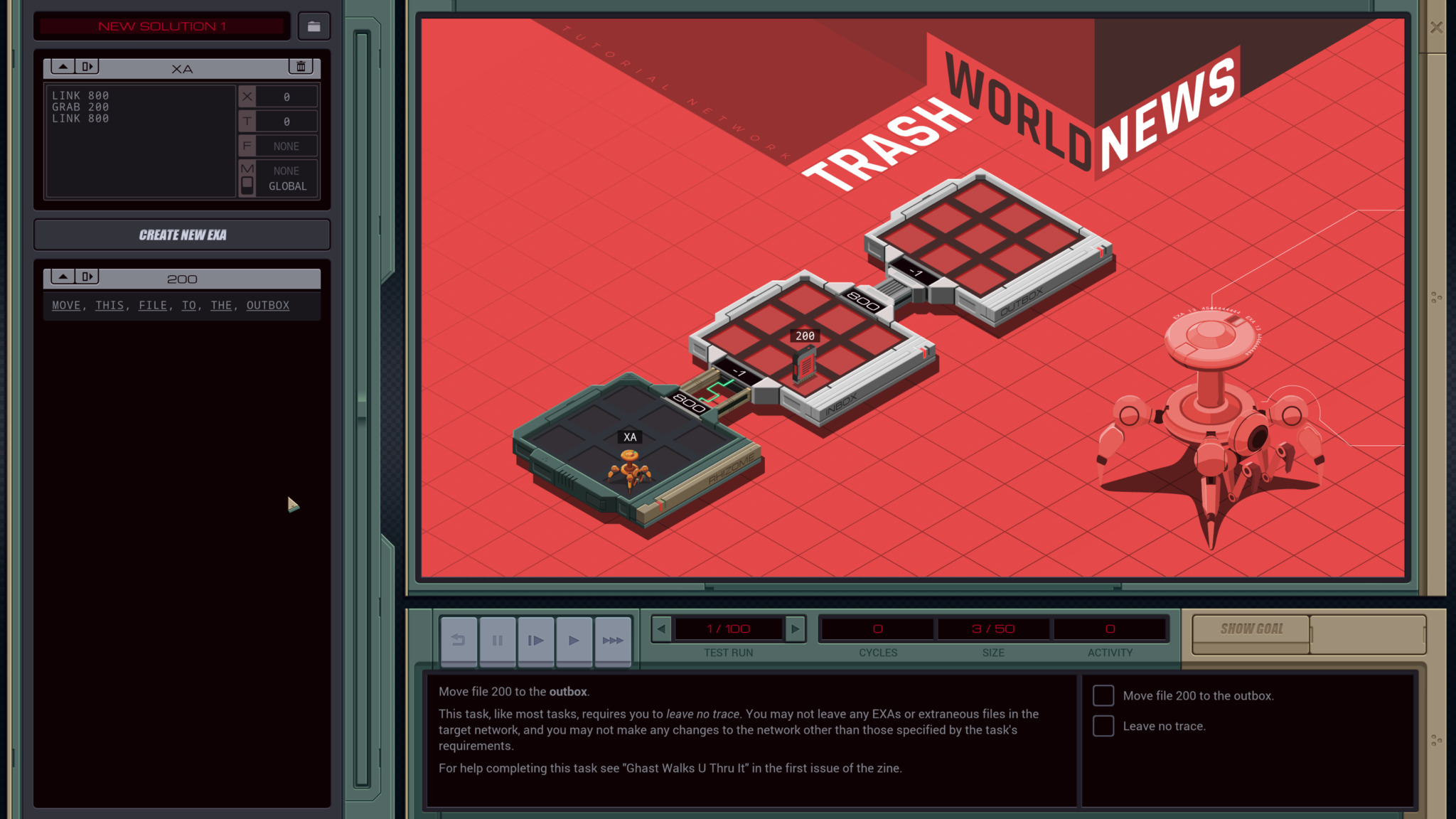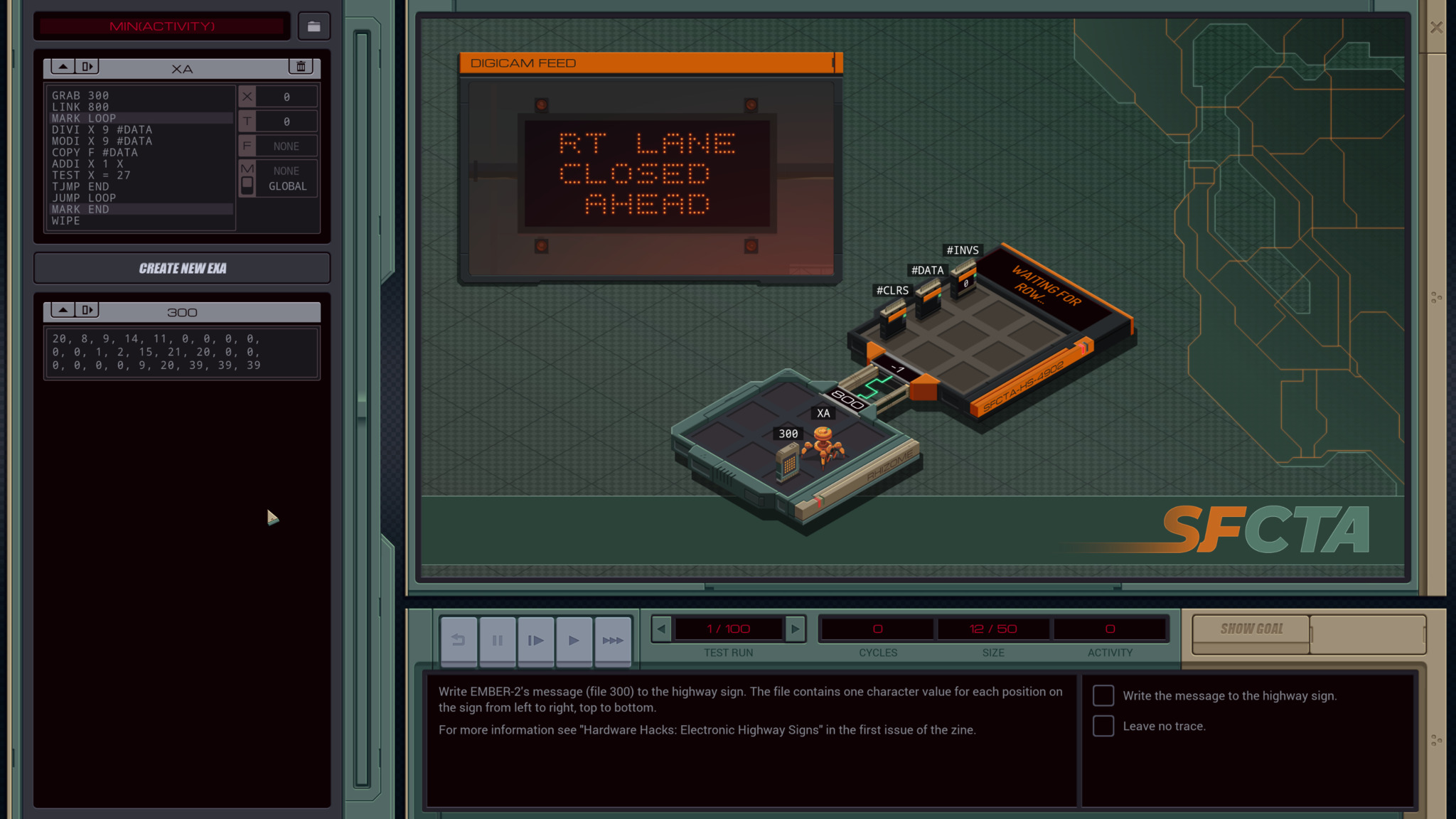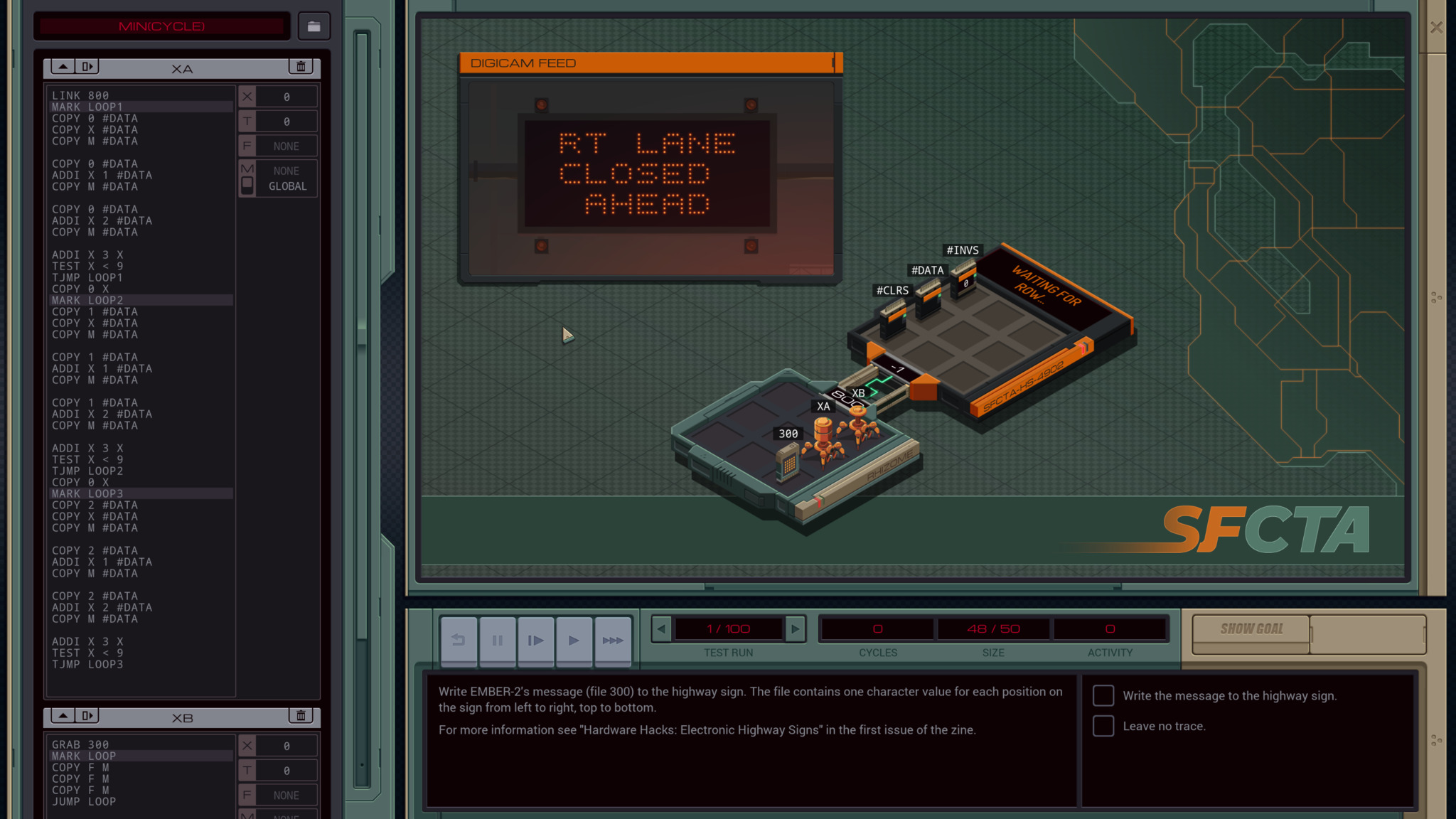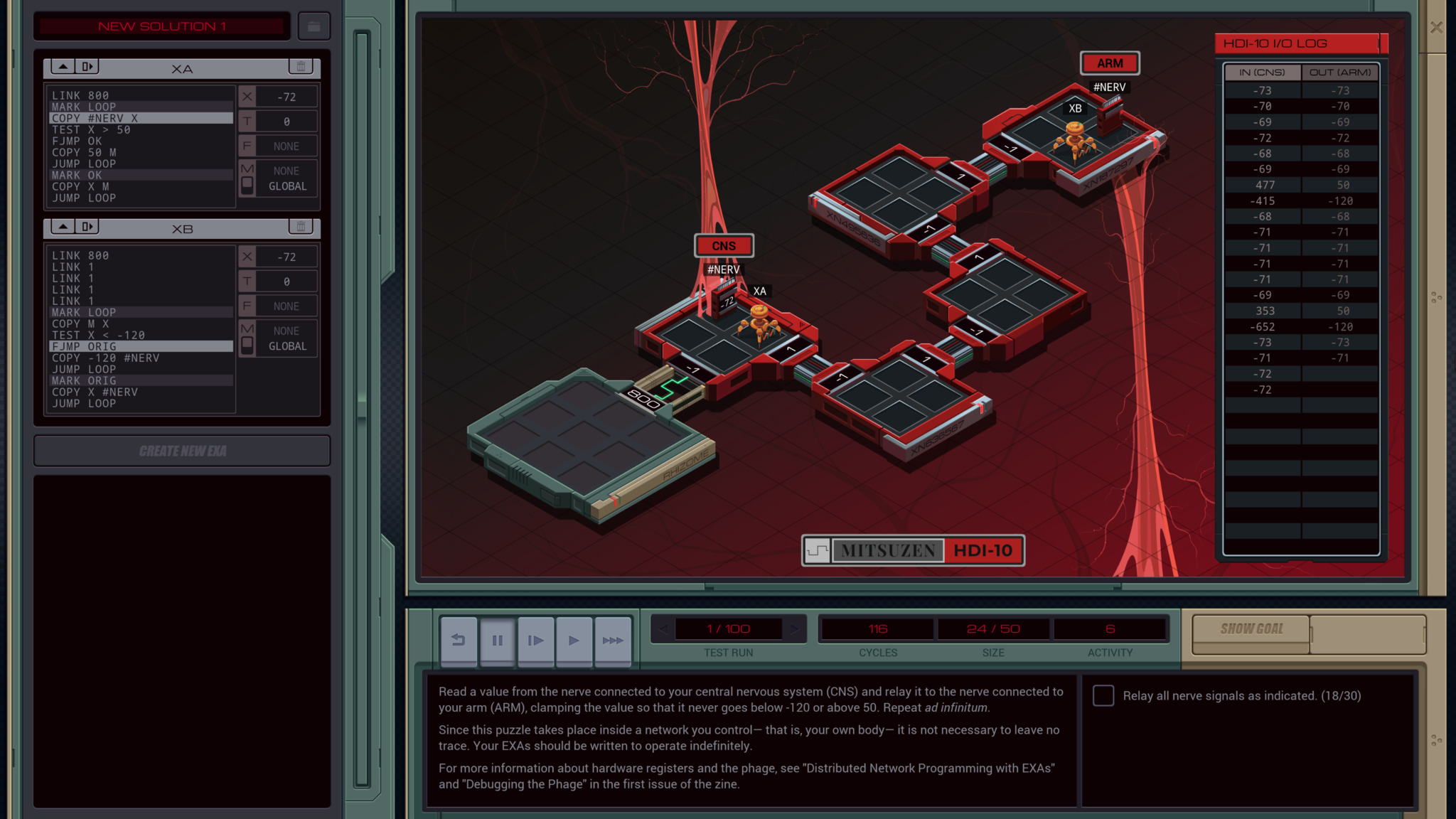Zachtronics’ new assembly game EXAPUNKS was released this week. This post covers some optimization techniques I discovered during play. This post contains spoilers but keep reading because this game is pretty boring by virtue of being similar to real programming.

First, some general guidelines for new players.

1. There are 3 metrics: speed, code size and exas.

2. You can only optimize one metric at a time.

3. Most optimizations are boilerplates(covered below).

4. Solve all the puzzles first. Optimization is a different game in itself. I solved all the puzzles before starting optimization.

5. When you are optimizing a loop, use all 32 lines.

# Technique 0: Omit DROP and HALT

You don’t need `DROP` or `HALT` on the last line. It’s kind of like how in Linux you don’t call `free()` right before program terminates.

Example: the first program.# Technique 1: ALU register output

Consider this program:

``````LINK 800
GRAB 200
COPY F X
MULI X F X
SUBI X F X
COPY X F
``````

Instead of saving the output of the ALU instructions to X register, just output to the final register F.

``````LINK 800
GRAB 200
MULI X F X
SUBI X F F
``````# Technique 2: Unroll Loops

This has nothing to do with SIMD. The goal is to minimize branch penalty. The idea is to make the loop body fatter so there are more action for every `TEST` instruction. It gets repetitive pretty fast but it’s unavoidable if you want to be on the leaderboard. Maybe one day Zachtronics will come up with a new scoring mechanism to circumvent this.

Note: before unwinding loops, try and eliminate as many instructions as possible.

## Example 1:

This is the tutorial puzzle where you write n down to 0. Here is an optimized version.

``````LINK 800
GRAB 200
COPY F X
WIPE
MAKE

MARK LOOP
DIVI X 13 T
FJMP DONE
COPY X F
@rep 13
SUBI X @{1,1} F
@end
JUMP LOOP

MARK DONE
COPY X F
@rep 11
MODI -1 X X
COPY X F
@end
``````

Note:

``````@rep 13
SUBI X @{1,1} F
@end
``````

expands to

``````SUBI X 1 F
SUBI X 2 F
SUBI X 3 F
SUBI X 4 F
SUBI X 5 F
SUBI X 6 F
SUBI X 7 F
...
SUBI X 13 F
``````

Basically, you pack the loop as tightly as you can(13 items) and then handle the remainder(12 items).

## Example 2, highway sign:

Naive version:``````GRAB 300
MARK LOOP
DIVI X 9 #DATA
MODI X 9 #DATA
COPY F #DATA
TEST X = 27
TJMP END
JUMP LOOP
MARK END
WIPE
``````

Unrolled version:# Technique 3: Quick halt

`MODI` packs subtraction and halting into one. It either subtracts 1 or throws a division by 0 runtime error equivalent to `HALT`.

Here is the formula for modulo:

``````let a = -1 in
r = a % n
= a - n * ⌊a / n⌋
= n - 1
``````

There are more in the runtime error section in the manual but MODI is the most useful one.

# Technique 4: Use `T` as Counter

`TEST` sets `T` to 1 if positive. So a common practice is to use `T` as a counter. When `T = 0` the loop will exit.

``````COPY 8 T
MARK LOOP

NOOP
SUBI T 1 T

TJMP LOOP
NOOP
``````

Alternatively, do something N times then die.

``````COPY <N> T
MARK LOOP
NOOP
MOD -1 X X
JUMP LOOP
``````

# Technique 5: Eliminate idle nodes

When a node is idling, ie. blocking read from channel, queuing to cross a link, the cycle counter is still ticking. So try `REPL`ing in a vacant host and fan out work load.

Example:# Technique 6: Reorder control flow to eliminate jumps

These are context specific. The hot path should be the one immediately after TEST to save a `JUMP`.

# Conclusion

In this post, I covered some common EXAPUNKS optimization techniques. If you have more please let me know!